# Writing an Inequality Given a Graph on the Number Line Online Quiz

#### Better Business Writing Skills

13 Lectures 1.5 hours

#### Freelance Writing 101: Start Your Successful Writing Career

25 Lectures 1 hours

#### Writing That Stands Apart

14 Lectures 1 hours

Following quiz provides Multiple Choice Questions (MCQs) related to Writing an Inequality Given a Graph on the Number Line. You will have to read all the given answers and click over the correct answer. If you are not sure about the answer then you can check the answer using Show Answer button. You can use Next Quiz button to check new set of questions in the quiz.Q 1 - Write an inequality for the graph on the number line given below.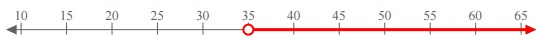### Explanation

Step 1:

From the given graph on the number line since the arrow is towards right and there is an open circle, it is a ‘greater than’ inequality.

Step 2:

Since it is starting from 35 with an open circle, the inequality is x > 35

Q 2 - Write an inequality for the graph on the number line given below.### Explanation

Step 1:

From the given graph on the number line since the arrow is towards left and there is a closed circle, it is ‘less than or equal to’ inequality.

Step 2:

Since it is starting from −20 with a closed circle, the inequality is x ≤ −20

Q 3 - Write an inequality for the graph on the number line given below.### Explanation

Step 1:

From the given graph on the number line since the arrow is towards left and there is an open circle, it is a ‘less than’ inequality.

Step 2:

Since it is starting from 190 with an open circle, the inequality is x < 190.

Q 4 - Write an inequality for the graph on the number line given below.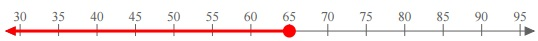### Explanation

Step 1:

From the given graph on the number line since the arrow is towards left and there is a closed circle, it is ‘less than or equal to’ inequality.

Step 2:

Since it is starting from 65 with a closed circle, the inequality is x ≤ 65.

Q 5 - Write an inequality for the graph on the number line given below.### Explanation

Step 1:

From the given graph on the number line since the arrow is towards left and there is a closed circle, it is ‘less than or equal to’ inequality.

Step 2:

Since it is starting from −15 with a closed circle, the inequality is x ≤ −15.

Q 6 - Write an inequality for the graph on the number line given below.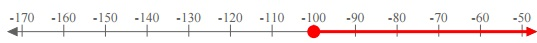### Explanation

Step 1:

From the given graph on the number line, since the arrow is towards right and there is a closed circle, it is ‘greater than or equal to’ inequality.

Step 2:

Since it is starting from −100 with a closed circle, the inequality is x ≥ −100.

Q 7 - Write an inequality for the graph on the number line given below.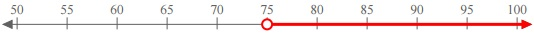### Explanation

Step 1:

From the given graph on the number line, since the arrow is towards right and there is an open circle, it is ‘greater than’ inequality.

Step 2:

Since it is starting from 75 with an open circle, the inequality is x > 75.

Q 8 - Write an inequality for the graph on the number line given below.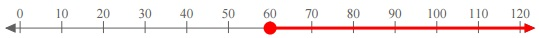### Explanation

Step 1:

From the given graph on the number line, since the arrow is towards right and there is a closed circle, it is ‘greater than or equal to’ inequality.

Step 2:

Since it is starting from 60 with a closed circle, the inequality is x ≥ 60.

Q 9 - Write an inequality for the graph on the number line given below.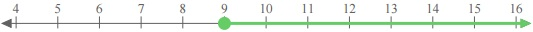### Explanation

Step 1:

From the given graph on the number line, since the arrow is towards right and there is a closed circle, it is ‘greater than or equal to’ inequality.

Step 2:

Since it is starting from 9 with a closed circle, the inequality is x ≥ 9.

Q 10 - Write an inequality for the graph on the number line given below.### Explanation

Step 1:

From the given graph on the number line since the arrow is towards left and there is an open circle, it is ‘less than’ inequality.

Step 2:

Since it is starting from 170 with an open circle, the inequality is x < 170

writing_inequality_given_graph_on_number_line.htm